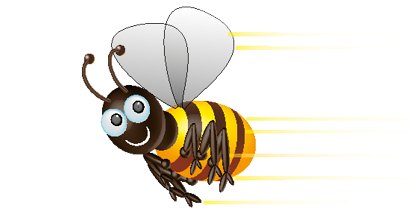HintWhat is the 6th coin in the pattern?

c

What is the 8th coin in the pattern?

c

What is the 6th coin in the pattern?

c

What is the 8th coin in the pattern?

c

What is the 6th coin in the pattern?

c

What is the 8th coin in the pattern?

c

What is the 4th coin in the pattern?

c

What is the 6th coin in the pattern?

c